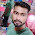### Program 312: Factorial of a number using Recursion

Program 312: Factorial of a number using Recursion

```#include<stdio.h>
int GetFactorial(int number);
main()
{
int number,factorial;
printf("Enter a number for knowing it's factorial\n");
scanf("%d",&number);
factorial=GetFactorial(number);
printf("%d!=%d\n",number,factorial);
}

int GetFactorial(int number)
{
if(number>0)
{
return number*GetFactorial(number-1);
}
else
{
return 1;
}
}```
Explanation:

1. This program starts with initializing :
• number → To store given number to get Factorial
• factorial →Used to store output
• int GetFactorial→ Declaration of function
2. ``` printf("Enter a number for knowing it's factorial\n");
scanf("%d",&number);```
To take input from user
3. `factorial=GetFactorial(number);`
Calling GetFactorail Function and received output from the called function(i.e GetFactorial) will be sored in factorial
4. ```int GetFactorial(int number)
{
if(number>0)
{
return number*GetFactorial(number-1);
}
else
{
return 1;
}
}```
Now lets take a number 4 to get factoraial of 4.
now number is greater than 0(i.e 4>0) is true so if part is executed
5. Now
1. return 4*GetFactorial(4-1);
2. GetFactorial(3)---> return 3*GetFactorial(3-1)
3. Substitute 3*GetFactorial(3-1) in Step 1
4. So Step 1 will be return 4*3*GetFactorial(3-1)
5. Now again GetFactorial(2)--->return 2*GetFactorial(2-1)
6. So Step 4 will be return 4*3*2*GetFactorial(2-1)
7. GetFactorial(1)--->return 1*GetFactorial(1-1)
8. So again Step 6 will be return 4*3*2*1*GetFactorial(1-1)
9. As GetFactorial(0)-->Since if(0>0) is false else part  will be executed.So it will return 1.
10. So Step 8  will be return 4*3*2*1*1
11. Finally it returns value of 4*3*2*1*1=24
6. factorial=24 and this will be printed as output

Output:

#### 1 comment:

1.factorial hundred In the last few days, the “factorial of 100” is one of the top subjects and a lot of maths geeks compute it using voice assistants such as Alexa, Shiri, etc.
factorial hundred In the last few days, the “factorial of 100” is one of the top subjects and a lot of maths geeks compute it using voice assistants such as Alexa, Shiri, etc.

 Donate Buy me a coffee \$2.00 USD Buy me a burger \$5.00 USD Buy me a pizza \$10.00 USDCompiler Used by me C-Free 5.0(Recommended) Other Compilers Code Blocks(Recommended) Online Compilers Ideone(Remember to give input before executing online where ever necessary)